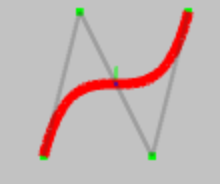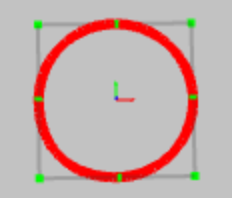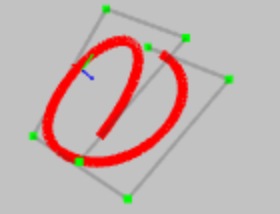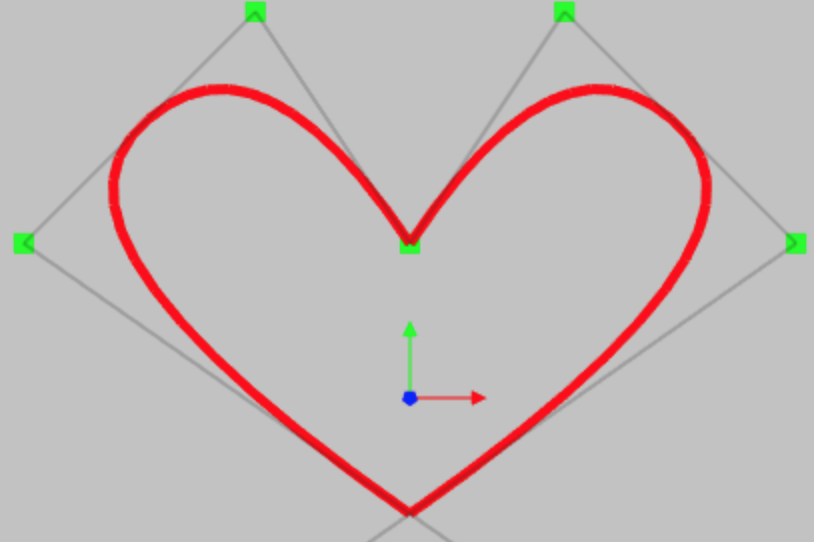#### I'm available to contribute to your next groundbreaking software development project. I have 12+ years of expertise in CG (computer graphics) software development with strong skills in mathematics and computational geometry. Recently I have acquired new skills like Flutter(Dart), firebase, angular, react, etc for full-stack mobile/web app development. Please contact me on my email id pawangami@gmail.com or refer me.

##### To know more about me, Visit my linkedin profile.

This WebGL based NURBS application will help you to understand the NURBS curves in a practical and intuitive way. You can also create your own curves and download it, as a text file. You can start with some predefined curves by clicking on one of the images and create new curves, by adding, deleting or modifying the curve parameters. Click here for more information.

• This website requires webGL enabled browsers, preferably Chrome. If you are using a browser other than Chrome, then check if webGL is enabled.

Please select a plane, by clicking one of the buttons - ‘XY Plane’, ‘YZ Plane’ or ‘ZX Plane’.

Enter u value between {{uMin}} and {{uMax}} :
u : {{sliderVal}} , Evaluated Point : {{evalPointText}}

Upload NURBS data :

Please login to save the model on the server File name already exists. The previous model will be deleted
Selection from a modal: {{ selected }}Rendering

Select the type of the curve

For Bezier curves the total number of control points are exactly equal to order of the curve, so to add/remove control points increase/decrease the degree. Bezier curves are always clamped.

Degree :

Control Points : x, y, z, w
{{k}} :

Knots :
Clamped at start Clamped at end

Update Spline Instantly

Number of segments :

## What is NURBS?

Non Uniform Rational B-Spline is a mathematical model to represent the free form curves and surfaces in computer graphics. It is mostly used in Computer Aided Design (CAD) and creating characters for video games. The mathematics behind NURBS is very vast and complex, but this nurbs-calculator covers only the evaluation part of NURBS curves. And I have tried to make it as simple as possible, so that anyone can understand NURBS without knowing the complex mathematics.

Evaluation means finding the coordinates of a point on the curve, corresponding to some parameter.

Here's is the short summary of each term of NURBS:

• NU - Non-Uniform - The knot vector can be uniform or non-uniform.
• R - Rational – If the control points have weights other than 1, then it is a rational curve/surface.
• BS - B-Splines - Defines the mathematical formula for the curve/surface.

Each of these terms is explained in detail here.

Who can use nurbs-calculator?
• Students who are learning geometric modelling and computer graphics. They can use it to understand the properties of NURBS curves, by creating some sample curves and modifying the existing curve parameters.
• CAD engineers, who designs and creates models of machine parts, using some CAD software. They can use nurbs-calculator as a small light-weight tool to understand the spline geometries in their designs.
• CAD software developers, can use it as a reference to visualize the curve from available NURBS data.
• Designers, who create characters for video games. They can use it to create some free-form curves.
• Anyone who is interested in geometric modelling and computer graphics.

Why do you need nurbs-calculator?
• Splines and freeform curves have a lot of usage in designing of machine part, ship building and gaming industry. There are a lot of CAD softwares in which you can create NURBS curves, like Autodesk Maya, Rhinoceros 3D and SolidWorks But these softwares require installation on desktop PC’s and consume a lot of computer resources. Also some of these softwares don’t allow users to change all the NURBS parameter. If you just want to work with NURBS curves and looking for a small tool, which gives you full control on all the curve parameters, then nurbs-calculator is for you. You can create new curves and modify the curve parameters from your mobile/ tablet/ laptop browser.
• If you are not new to NURBS, then you might have read the theory of NURBS from this website - http://www.cs.mtu.edu/~shene/COURSES/cs3621/NOTES/ . This is a very good course to start, but the problem is that you can’t understand all the properties of NURBS, until you do a practical, by creating some curves and modifying the parameters. To do this either you have to write your own programs or use some commercial CAD softwares, which is a lot of overhead, just to understand the NURBS. In this case nurbs-calculator will be very useful for you.

What are the terminologies?
##### Curve parameters:

A NURBS curve can be created by just 3 parameters – degree, control point and knot vector. The term knot vector is very confusing. Actually it’s just a sequence of some real numbers, in non-decreasing order.

##### Degree and order:

It is the measure of curvature of the curve. A curve with degree 1 is called as linear curve, with degree 2 is called as quadratic and degree 3 is called as cubic. In most of NURBS applications you will find curves of degree 2 and 3, only. The degree of the curve is integer and should be > 0. Order of the curve = degree + 1.

##### Control points:

The control points determine the shape of the curve. Each point is having 4 float values – x position, y position, z position and weight of the control point. The minimum number of control points required to render a NURBS curve is the same as the order of the curve. So you need atleast 4 control points for degree 3 curve.

##### U parameter:

In mathematics, the equation of a curve can be expressed as a function of an independent variable, called as a parameter. In nurbs-calculator I call this parameter as u parameter. U parameter is used to calculate the coordinates of a point, on the curve, from its parametric equation. Generally it is assumed that u is 0 at the start of the curve and 1 at the end. So at middle of the curve the value of u will be 0.5. Let's assume that there is a line starting at (1,1) and ending at (2,2). Also assume that u=0 at start and u=1 at end. If you are asked to find the coordinates of point, which lies at middle of the line, then in mathematical term you will say that what should be coordinates of point at u=0.5. You can provide this u value to the parametric equation of line and calculate the coordinates at middle point.You can also assume that u=1 at start and u=2 at end or u=10 at start and u=100 at end. It is your assumptions which defines the middle point of the curve, so for 0< u < 1, middle point is at u = 0.5, for 1 < u < 2, middle is at u = 1.5 and for 10 < u < 100 middle is at u=55.

##### Knot vector:

As mentioned earlier, knot vector is a sequence of some real numbers, in non-decreasing order. This sequence depends on the assumption of u values. If you are assuming that u varies from 0 to 1, then knot vector is sequence of numbers, between 0 and 1. So basically knot values are u values. There is no significance of each knot value separately. It is the sequence, which is important. The total number of knots = number of control points + degree + 1. If degree of the curve is 2 and there are 4 control points, then there will be total 7 knot values. A curve will be called as Non-Uniform, if the sequence of these knot values is non-uniform (e.g 0.0, 0.4, 0.5, 0.6, 0.9, 0.95, 1.0 ) and called as uniform if this sequence is uniform (e.g. 0.0, 0.167, 0.333, 0.5, 0667, 0.833, 1.0).

##### Knot multiplicity:

Multiplicity means repetition of knot values. Multiplicity is a property of knots that refers to the number of control points associated to a knot. On a cubic curve (degree 3), a knot can have a multiplicity of 1, 2, or 3, but not more than 3. Similarily for degree 2 curve the multiplicity can’t be more than 2. If knot values have multiplicity = degree + 1 at the start, then curve will be clamped at start, that means the curve touches the first control point. If the multiplicity < (degree+ 1) at start, then the curve will not touch the first control point and the curve will start at u = k[p], where p=degree. Same is applicable for the end knots.If the multiplicity at the end is < (degree + 1), then the curve will not touch the last control point and curve will end at u=k[m-p], where m = (total number of knots - 1). That means, if knot vector is = {0, 0.111, 0.222, 0.333, 0.445, 0.556, 0.667, 0.778, 0.889, 1.0} for degree 2 curve, then the curve will start from u = 0.2, which is k and end at u=0.778, which is k. If the multiplicity > (degree + 1), then the curve will split in disjoint parts and the control point will be unused.

These terminolgies have been explained in simple way and may not represent the exact definition. To understand these terms in details, please visit CS3621 Introduction to Computing with Geometry Course Notes or read The NURBS Book. NURBS and its properties are also explained on Rhinoceros - NURBS and NURB curves - a guide for the uninitiated .

How to use nurbs-calculator?
• Move your mouse over any of the help button and it will display the information about that element or move the mouse directly over the element like webGL window.
• Click on the one the images on the left side and the curve will be rendered in the webGL window at right side.
• To rotate the curve, click left mouse button in the webGL window and drag. To zoom in/out, scroll mouse wheel. If curve goes out of visible area, then press ‘Zoom to fit’ button, below the webGL window.
• If you want to view the curve from front, then press XY plane, for side view click YZ plane and for top view click on ZX plane.
• You can increase or decrease the degree of the curve. Please note that it should be an integer and should be > 0.
• There are 4 edit boxes for each control point – first is for x value, second for y value, third for z value and last is for weight of the control point. You can add/remove as many control point as you want, but the total number of control points should not be less than order of the curve. To add a control point click on the add button. It will create a control at some random position. You can modify a control point (shown in green color) by selecting and dragging it in the webGL window.
• Modify control point – This is the default option. Select and drag any of the control points (shown in green color) in the webGL window, to modify its position.
• Add a control point – Select this option and choose a plane by clicking appropriate button ( i.e.’XY Plane, ‘YZ Plane’ or ‘ZX Plane’ ), then click in webGL window to add a control point at that position. A control point can also be added by clicking on the ‘Add’ button on the left side, but this will add the control point at some random position.
• Remove a control point – Select this option and click on any of the control point to remove it.
• Remember to switch back to ‘Modify control point’ option, once you are done with adding/removing the control points, otherwise it will keep on adding/removing.
• If you want that the curve should touch the first control point, then the checkbox ‘clamped at start’ should be checked. Same is applicable for last control point. If the curve is clamed at start, then the first (degree+1) knots will be read only.
• To evaluate the point on the curve, move the slider, which is below the webGL window. It will display the u parameter and its corresponding point. You can also enter u parameter value in the edit box.
• To download the curve data, as a JSON click on ‘Download NURBS data’ and save it as text file.
• You can change the values of curve parameters in the downloaded JSON and upload it back, by clicking of ‘Choose file’ button below 'Upload NURBS data’.

Bézier curves, B-Splines and NURBS

NURBS is a generic term and Bézier curves and B-splines are specific cases of NURBS. As mentioned earlier you need only 3 parameters to render a NURBS curve - degree, control points and knot vector. Putting some restrictions on these 3 parameters can create Bézier curves and B-splines.

• Bézier curves:
1. The number of control points in Bézier curves is exactly equal to (degree + 1). That means for degree 2 curve, there will be 3 control points. If we add 1 control point, then the degree will also increase by 1. This is the main limitation of Bezier curves. Sometimes we want to add more control point at some portion of the curve (to precisely control the shape of that portion), but if degree is increased, then it will affect the shape of entire curve.
2. Since the number of control points and degree are fixed, so according to the formula –> k = n+p+1, the number of knots will always be 2*(degree+1).
3. It is always clamed at start and end. That means the curve is tangent at the first and last control point.
4. Since it is clamped at start and end, so first degree+1 knot values will be 0 and last degree+1 knot values will be 1. That means the knot vector of Bezier curve doesn’t contains any value other than 0 and 1.
5. It may be a rational curve, means all the control points can have different weights
• B-Splines:
1. Minimum number of control points = degree + 1. More control points can be added without increasing the degree
2. Knots values can be uniform or non-uniform.
3. Curve may or may not be clamed at end points. That means the curve may or may not touch the end points.
4. It is always irrational, so all the control points should have equal weights, usually 1.
• NURBS: All the properties are same as b-splines, except that it considers the weight of the control points. So each control point can have different weight. That is why it is called as rational b-splines.

## What is WebGL?

WebGL (Web Graphics Library) is a JavaScript API for rendering interactive 3D computer graphics and 2D graphics within any compatible web browser (reference - wiki - webGL). In nurbs-calculator I have used three.js to render the nurbs curves, so that a curve can be viewed in 3D. Three.js is a cross-browser JavaScript library/API used to create and display animated 3D computer graphics in a web browser (reference - wiki - three.js). Three.js uses WebGL.